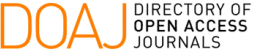### Sufficient Conditions for Existence of the LU Factorization of Toeplitz Symmetric Tridiagonal Matrices

C. G. Almeida, S. A. E. Remigio

#### Abstract

The characterization of inverses of symmetric tridiagonal and block tridiagonal matrices and the development of algorithms for finding the inverse of any general non-singular tridiagonal matrix are subjects that have been studied by many authors. The results of these research usually depend on the existence of the LU factorization of a non-sigular matrix A, such that A = LU. Besides, the conditions that ensure the nonsingularity of A and its LU factorization are not promptly obtained. Then, we are going to present in this work two extremely simple sufficient conditions for existence of the LU factorization of a Toeplitz symmetric tridiagonal matrix A. We take into consideration the roots of the modified Chebyshev polynomial, and we also present an analysis based on the parameters of the Crout’s method.

#### Keywords

Toeplitz tridiagonal matrix, Crout's method, tridiagonal and diagonally dominant matrix

PDF

#### References

R. L. Burden and J. D. Faires}, Numerical Analysis, 9th ed., Springer, New York, USA, 2014.

P. Z. Revesz, Cubic spline interpolation by solving a recurrence equation instead of a tridiagonal matrix, Proceedings of the First International Conference on Mathematical Methods and Computational Techniques in Science and Engineering, WSEAS Press, 2014, Athens, Greece, 21--25.

P. Z. Revesz, Introduction to Databases: From Biological to Spatio-Temporal, 2010, Springer, New York, USA.

M. E. A. El-Mikkawy, Notes on linear systems with positive definite tridiagonal coefficient matrices, Indian Journal of Pure and Applied Mathematics, vol. 33, 8, 1285 - 1293, 2002.

M. E. A. El-Mikkawy, On the inverse of a general tridiagonal matrix, Applied Mathematics and Computation, vol. 150, 3, 669 - 679, 2004, issn: 0096-3003, doi: https://doi.org/10.1016/S0096-3003(03)00298-4.

M. El-Mikkawy and A. Karawia, Inversion of general tridiagonal matrices, Applied Mathematics Letters, vol. 19, 712 - 720, 2006, doi: 10.1016/j.aml.2005.11.012.

C. F. Fischer and R. A. Usmani, Properties of some Tridiagonal Matrices and their application to Boundary Value

Problems, SIAM Journal on Numerical Analysis, vol. 6, 1, 127 - 142, 1969, url: https://www.jstor.org/stable/2156523.

G. Meurant, A review on the inverse of symmetric tridiagonal and block tridiagonal matrices, SIAM Journal Matrix Anal. Appl., vol. 13, 3, 707 - 728, 1992.

R. E. Bank and D. J. Rose, Marching algorithms for elliptic boundary value problems. I: The constant coefficient case, SIAM Journal on Numerical Analysis, vol. 14, 5, 792 - 829, 1977.

C. M. da Fonseca and J. Petronilho, Explicit inverses of some tridiagonal matrices, Linear Algebra and its Applications, vol. 325, 7 - 21, 2001.

Xi-Le Zhao and Ting-Zhu Huang, On the inverse of a general pentadiagonal matrix, Applied Mathematics and Computation, vol. 202, 2, 639 - 646, 2008, issn: 0096-3003, doi: https://doi.org/10.1016/j.amc.2008.03.004.

J. M. McNally, A fast algorithm for solving diagonally dominant symmetric pentadiagonal Toeplitz systems, Journal of Computational and Applied Mathematics, vol. 234, 4, 995 - 1005, 2010, issn: 0377-0427, doi: https://doi.org/10.1016/j.cam.2009.03.001.

S. S. Askar and A. A. Karawia, On Solving Pentadiagonal Linear Systems via Transformations, Mathematical Problems in Engineering, George S. Dulikravich, Hindawi Publishing Corporation, vol. 2015, 2015,

doi: https://doi.org/10.1155/2015/232456.

J. M. McNally and L. E. Garey and R. E. Shaw, A split-correct parallel algorithm for solving tridiagonal symmetric Toeplitz systems, Int. J. Comput. Math., vol. 75, 303--313, 2000.

DOI: https://doi.org/10.5540/tcam.2022.024.01.00177

### Refbacks

• There are currently no refbacks.

Trends in Computational and Applied Mathematics

A publication of the Brazilian Society of Applied and Computational Mathematics (SBMAC)

Indexed in: